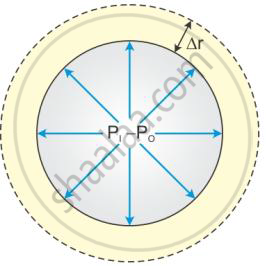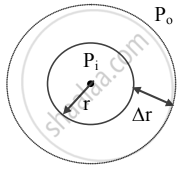# Derive Laplace’S Law for Spherical Membrane of Bubble Due to Surface Tension. - Physics

Derivation
Sum

Derive Laplace’s law for spherical membrane of bubble due to surface tension.

Derive Laplace’s law for a spherical membrane.

#### Solution 1

Consider a spherical liquid drop and let the outside pressure be Po and inside pressure be Pi, such that the excess pressure is Pi − PLet the radius of the drop increase from r to Δr, where Δr is very small, so that the pressure inside the drop remains almost constant.

Initial surface area (A1) = 4Πr2

Final surface area (A2) = 4Π(r + Δr)2

= 4π(r2 + 2rΔr + Δr2)

= 4Πr2 + 8ΠrΔr + 4ΠΔr2

As Δr is very small, Δr2 is neglected (i.e. 4πΔr2≅0)

Increase in surface area (dA) =A2 - A1= 4Πr2 + 8ΠrΔr - 4Πr2

Increase in surface area (dA) =8ΠΔr

Work done to increase the surface area by 8ΠrΔr is extra energy.

∴ dW = TdA

∴ dW = T*8πrΔr         .......(Equ.1)

This work done is equal to the product of the force and the distance Δr.

dF=(P1 - P0)4πr

The increase in the radius of the bubble is Δr.

dW = dFΔr = (P1 - P0)4Πr2*Δr  ..........(Equ.2)

Comparing Equations 1 and 2, we get

(P1 - P0)4πr2*Δr = T*8πrΔr

∴(P_1 - P_0) = (2T)/R

This is called Laplace’s law of spherical membrane.

#### Solution 21. The free surface of drops or bubbles is spherical in shape.
Let,
Pi = inside pressure of a drop or air bubble
Po = outside pressure of the bubble
r = radius of drop or bubble.
2. As drop is spherical, Pi > Po
∴ excess pressure inside drop = Pi − P
3. Let the radius of drop increase from r to r + ∆r so that inside pressure remains constant.
4. Initial area of drop A1 = 4πr2 ,
Final surface area of drop A2 = 4π (r + ∆r)
Increase in surface area,
∆A = A2 − A1 = 4π[(r + ∆r)2 − r2]
= 4π[r2 + 2r∆r + ∆r2 − r2]
= 8πr∆r + 4π∆r2
5. As ∆r is very small, the term containing ∆r2 can be neglected.
∴ dA = 8πr∆r
6. Work is done by a force of surface tension,
dW = TdA = (8πr∆r)T ….(1)
This work done is also equal to the product of the force F which causes an increase in the area of the bubble and the displacement Δr which is the increase in the radius of the bubble.
∴ dW = FΔr
The excess force is given by,
(Excess pressure) × (Surface area)
∴ F = (Pi – Po) × 4πr
∴ dF = (Pi – Po)A
dW = F∆r = (Pi − Po) A∆r
From equation (1),
(Pi − Po) A∆r = (8πr∆r) T
∴ Pi − Po = (8pirDeltarT)/(4pir^2Deltar) ........(∵ A = 4πr2)
∴ Pi − Po = (2T)/r ….(2)

Equation (2) represents Laplace’s law of spherical membrane.

Concept: Surface Tension
Is there an error in this question or solution?